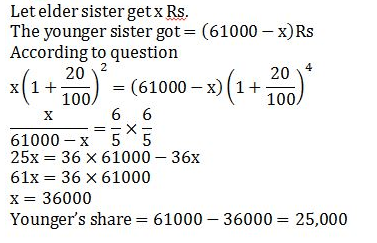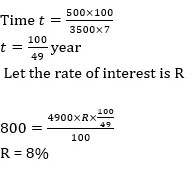# Quant (Simple & compound interest) Quiz for SBI PO PRE 2019 | 21th January 2019

Quant Quiz to improve your Quantitative Aptitude & Data Interpretation. For upcoming exams like Bank, SSC, Railway, UPSC, UPSSSC, CDS, UPTET, KVS, DSSSB and other Government exams.

Q1. Madhu invested a certain amount at the rate of 8 p.c.p.a. for 5 years and obtained a simple interest of Rs 3800. Had she invested the same amount at the same rate of interest for 2 years, how much amount would she have obtained as compound interest at the end of 2 years?

A) 1580.80

B) 1520

C) 1550.50

D) 1550

E) None of these

Q2. The RBI lends a certain amount to the SBI on simple interest for two years at 20%. The SBI gives this entire amount to Bharti Telecom on compound interest for two years at the same rate annually. Find the percentage earning of the SBI at the end of two years on the entire amount.

(a) 4%

(b) 3(1/7)%

(c) 3(2/7)%

(d) 3(6/7)%

(e) None of these

Q3. A mother divided an amount of Rs. 61,000 between her two daughters aged 18 years and 16 years respectively and deposited their shares in a bond. If the interest rate is 20% compounded annually and if each received the same amount as the other when they attained the age of 20 years, their shares are

(a) Rs. 35,600 and Rs. 25,400

(b) Rs. 30500 each

(c) Rs. 24,000 and Rs. 37000

(d) Rs. 36000  and Rs 25,000

(e) None of these

Q4. A sum of money lent at compound interest for 2 years at 20% per annum would fetch Rs 723 more, if the interest was payable half-yearly than if it was payable annually. The sum is?

(a) Rs. 38,000

(b) Rs. 30,000

(c) Rs. 20,000

(d) Rs. 25,000

(e) None of these

Q5. Manish borrowed some money at the rate of 7 per cent per annul for the first three years, 9 per cent per annul for the next six years and 10 per cent per annul for the period beyond nine years. If the total interest paid by him at the end of fifteen years is Rs 4050, how much money did he borrow( if simple interest be reckoned)?

(a) Rs 2800

(b) Rs 3600

(c) Rs 3000

(d) Rs 3500

(e) None of these

Q6. A man borrows Rs. 4000 at 20% compound rate of interest. At the end of each year he pays back Rs. 1500. How much amount should he pay at the end of the third year to clear all his dues ?

(a) Rs. 2592

(b) Rs. 2852

(c) Rs. 2952

(d) Rs. 2953

(e) None of these

Q7. Ashok borrowed some money at simple interest at the rate of 6 per cent per annul for the first two years, at the rate of 9 per cent per annul for the next three years and at the rate of 14% per cent per annul for the period beyond five years. If he pays a total interest of Rs. 11400 at the end of 9 years how much money did he borrow ?

(a) Rs. 16,000

(b) Rs. 14,000

(c) Rs. 18,000

(d) Rs. 12,000

(e) None of these

Q8. On Rs 3500 invested at a simple interest rate 7 per cent per annum, Rs 500 is obtained as interest in certain years, In order to earn Rs 800 as interest on Rs 4900 in the same number of years, what should be the rate of simple interest?

(a) 9%

(b) 10%

(c) 12%

(d) 8%

(e) None of these

Q9. A person invested a certain amount at simple interest at the rate of 6 per cent per annul earning Rs. 900 as an interest at the end of three years. Had the interest been compounded every year, how much more interest would he have earned on the same amount with the same interest rate after three years ?

(a) Rs. 38.13

(b) Rs. 25.33

(c) Rs. 55.08

(d) Rs. 35.30

(e) None of these

Q10. If Rs.1000 be invested at interest rate of 5% and the interest be added to the principle every 10 years, then In how many years  it will amount to Rs. 2000 ?# Solutions

Q1. Ans(a)Q2. Ans(a)Q3. AnsQ4.  Ans(b)S5. Ans(c)Q6. Ans(c)Q7. Ans(d)Q8. Ans()S9. Ans(c)S10. Ans(a)## WhatsApp Group Join here

Mail us at : ambitiousbaba1@gmail.com

3